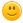# What is 90\$ -60\$.

110 views

What is 123-56-4 and what is 44+78.Thank you!

asked Mar 24, 2013 in Math

+1 vote

It is 63 for the first one. (123-56-4)Just do two numbers at a time.

And for the 2nd one it is 122. (44+78) Just add them as if they were single digits.

I can buy you a calculator for you if you want!Hopefully this helps.

Then 90-60 is 30 dollars.

answered Mar 24, 2013 by (37,210 points)
edited Apr 2, 2017
+1 vote
\$90 - &60 is \$30.

123 - 5 - 4 is 114.

44 + 78 is 122.

Hope it helps.

-CraftyGirlJuJu
answered Mar 24, 2013 by (15,270 points)
edited Mar 25, 2013
Sorry, CraftyGirlJuJu, you should check your math for the last two problems.  44 +78 is 122. But, it's okay, you were only one off!
Thanks, I guess in checking it on a calculator wasn't so great. I had 122 and then, I checked with a calculator and I got 121! Thanks so much! I'll edit that.
She stated that 44 + 78 is 122 smile828
+1 vote
123-56-4=63 and 44+78=122 hope this helps!
answered Mar 27, 2013 by (27,600 points)
it's a little problem but \$90-\$30
44

+78

_____

122

So do 40+70=110

then 4+8=12

then go add it

so it's 122

then  \$90-\$60=

thats 30 dollars

9-6=3 then add 0

123-56-4

123

-56

-4

____

123-60= 63
answered Jul 21, 2014 by (223,940 points)